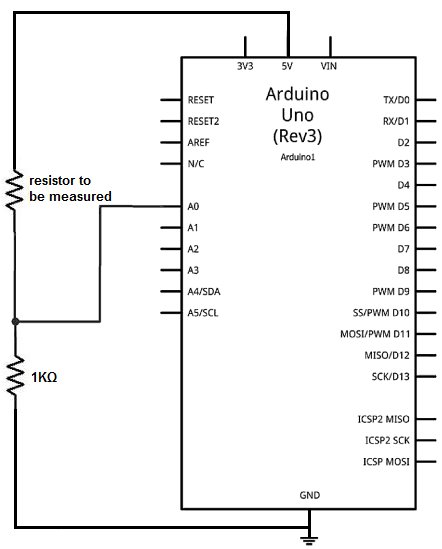# How to Build an Ohmmeter Using an Arduino

In this project, we will show how you can build an ohmmeter using an arduino.An ohmmeter is a device that can measure resistance.
Most of the times now, ohmmeters aren’t standalone devices but appear as one of type of measuring device amongst many in a multimeter. An advanced multimeter, today, would have an ohmmeter, voltmeter, ammeter, capacitance meter, continuity tester, and possibly a temperature sensor. For this circuit, we are only going to build an ohmmeter, as to measure resistance.
Using the correct hardware interface and uploaded code, we can turn the arduino into a device that can measure resistance.

### Parts Needed

• Arduino
• 1KΩ resistor
• Resistor (whose value you want to measure)

These are all the parts which you will need. The circuit is very basic, as you will see below.

### Circuit Schematic

The circuit schematic for the ohmmeter using an arduino is shown below.How this ohmmeter circuit works is that the 2 resistors in series forms a voltage divider circuit. One end of the resistor pair is hooked up to 5V and the other end is hooked up to ground. The 5 volts that the arduino provides gets divided up between the 2 resistors, depending on the value of the 2 resistors. The resistor which holds the greater resistance gets more of the voltage, according to ohm’s law formula, V=IR. The voltage that falls across a component is directly proportional to the amount of resistance it contains. Using this principle, we can set up a mathematical model to determine the resistance, based on the voltage division.

For more detail: How to Build an Ohmmeter Using an Arduino